# Class 8 RD Sharma Solutions – Chapter 8 Division Of Algebraic Expressions – Exercise 8.4 | Set 2

• Last Updated : 30 Apr, 2021

### Question 19. Divide 30x4 + 11x3 – 82x2 – 12x + 48 by 3x2 + 2x – 4

Solution:

We have to divide 30x4 + 11x3 – 82x2 – 12x + 48 by 3x2 + 2x – 4

So by using long division method we get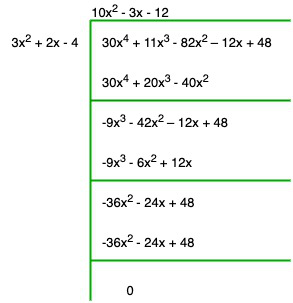Quotient = 10x2 – 3x – 12

Remainder = 0

### Question 20. Divide 9x4 – 4x2 + 4 by 3x2 – 4x + 2

Solution:

We have to divide 9x4 – 4x2 + 4 by 3x2 – 4x + 2

So by using long division method we get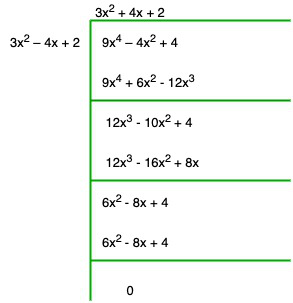Quotient = 3x2 + 4x + 2

Remainder = 0

### (i) Dividend = 14x2 + 13x – 15, Divisor = 7x – 4

Solution:

Dividing the Dividend by divisor, we get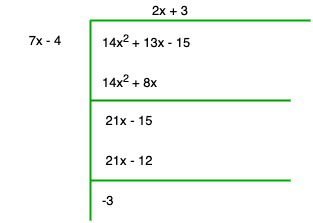Quotient = 2x + 3

Remainder = -3

### (ii) Dividend = 15z3 – 20z2 + 13z – 12, Divisor = 3z – 6

Solution:

Dividing the Dividend by divisor, we get

33z – 66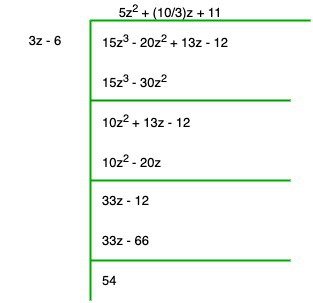Quotient = 5z2 + (10/3)z + 11

Remainder = 54

### (iii) Dividend = 6y5 – 28y3 + 3y2 + 30y – 9, Divisor = 2y2 – 6

Solution:

Dividing the Dividend by divisor, we get

3y2 – 9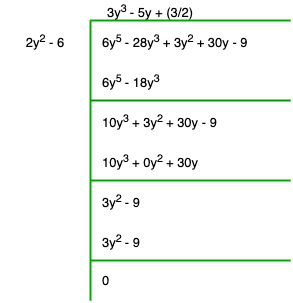Quotient = 3y3 – 5y + (3/2)

Remainder = 0

### (iv) Dividend = 34x – 22x3 – 12x4 – 10x2 – 75, Divisor = 3x + 7

Solution:

Dividing the Dividend by divisor, we get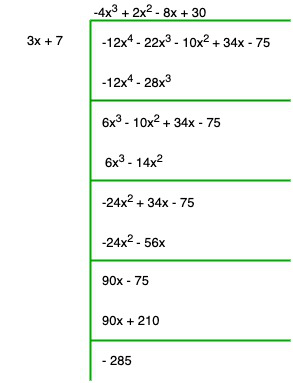Quotient = -4x3 + 2x2 – 8x + 30

Remainder = -285

### (v) Dividend = 15y4 – 16y3 + 9y2 – (10/3)y + 6, Divisor = 3y – 2

Solution:

Dividing the Dividend by divisor, we get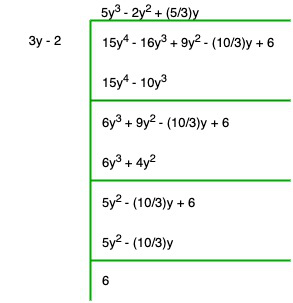Quotient = 5y3 – 2y2 + (5/3)y

Remainder = 6

### (vi) Dividend = 4y3 + 8y + 8y2 + 7, Divisor = 2y2 – y + 1

Solution:

Dividing the Dividend by divisor, we get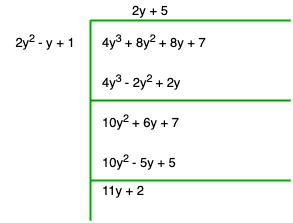Quotient = 2y + 5

Remainder = 11y + 2

### (vii) Dividend = 6y5 + 4y4 + 4y3 + 7y2 + 27y + 6, Divisor = 2y3 + 1

Solution:

Dividing the Dividend by divisor, we get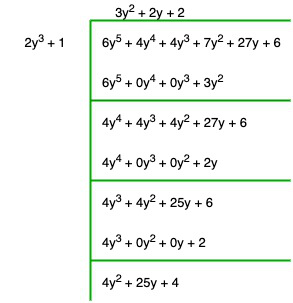Quotient = 3y2 + 2y + 2

Divisor = 4y2 + 25y + 4

### Question 22. Divide 15y4 + 16y3 + (10/3)y – 9y2 – 6 by 3y – 2 . Write down the coefficients of the terms in the quotient.

Solution:

We have to divide 15y4 + 16y3 + (10/3) y – 9y2 – 6 by 3y – 2

So by using long division method we get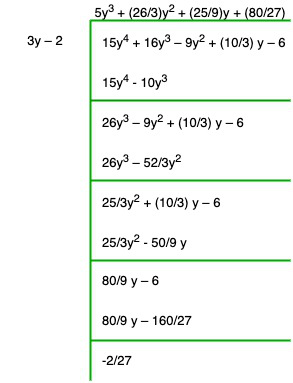Quotient = 5y3 + (26/3)y2 + (25/9)y + (80/27)

Remainder = (-2/27)

Co-efficient of y3 is 5

Co-efficient of y2 is 26/9

Co-efficient of y is 25/9 and,

Constant term = 80/27

### (i) x + 6 is a factor of x2 – x – 42

Solution:

Dividing x2 – x – 42 by x + 6, we get

-7x – 42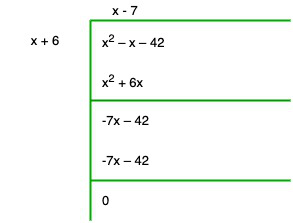Remainder = 0

Therefore, x + 6 is a factor of x2 – x – 42

### (ii) 4x – 1 is a factor of 4x2 – 13x – 12

Solution:

On dividing 4x2 – 13x – 12 by 4x – 1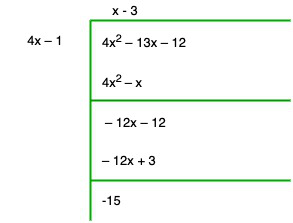Remainder = -15

Therefore, 4x-1 is not a factor of 4x2 – 13x – 12

### (iii) 2y – 5 is a factor of 4y4 – 10y3 – 10y2 + 30y – 15

Solution:

On dividing 4y4 – 10y3 – 10y2 + 30y – 15 by 2y – 5, we get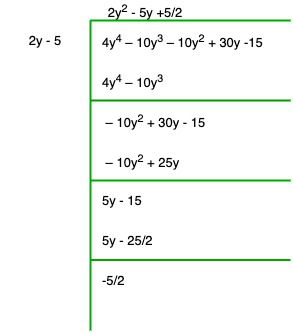Remainder = -5/2

Therefore, 2y – 5 is not a factor of 4y4 – 10y3 – 10y2 + 30y – 15

### (iv) 3y2 + 5 is a factor of 6y5 + 15y4 + 16y3 + 4y2 + 10y – 35

Solution:

On dividing 6y5 + 15y4 + 16y3 + 4y2 + 10y – 35 by 3y2 + 5, we get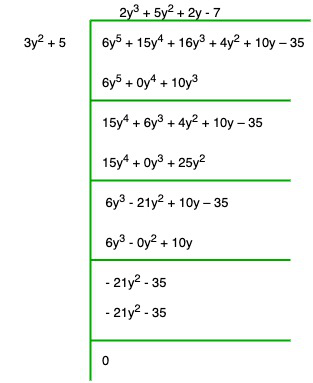Remainder = 0

Therefore, 3y2 + 5 is a factor of 6y5 + 15y4 + 16y3 + 4y2 + 10y – 35

### (v) z2 + 3 is a factor of z5– 9z

Solution:

On dividing z5 – 9z by z2 + 3, we get

-3z3 – 9z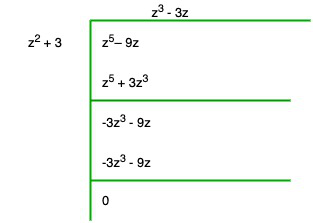Remainder = 0

Therefore, z2 + 3 is a factor of z5– 9z

### (vi) 2x2 – x + 3 is a factor of 6x5– x4 + 4x3 – 5x2 – x – 15

Solution:-

On dividing 6x5 – x4 + 4x3 – 5x2 – x – 15 by 2x2 – x + 3

-10x2 + 5x – 15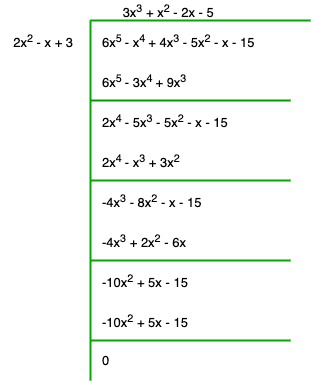Remainder = 0

Therefore, 2x2 – x + 3 is a factor of 60x5 – x4 + 4x3 – 5x2 – x – 15

### Question 24. Find the value of ‘a’, if x + 2 is a factor of 4x4 + 2x3 – 3x2 + 8x + 5a.

Solution:

Given that, x + 2 is a factor of 4x4 + 2x3 – 3x2 + 8x + 5a,

On dividing 4x4 + 2x3 – 3x2 + 8x + 5a by x + 2, we get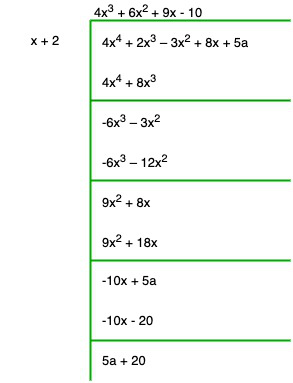Remainder = 5a + 20

5a + 20 = 0

a = -4

### Question 25. What must be added to x4 + 2x3 – 2x2 + x – 1 so that the resulting polynomial is exactly divisible by x2 + 2x – 3.

Solution:

On dividing x4 + 2x3 – 2x2 + x – 1 by x2 + 2x – 3, we get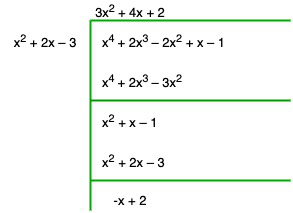Remainder = 0

The No. added to given polynomial to get remainder 0 will be :

x + 2 = 0

My Personal Notes arrow_drop_up
Recommended Articles
Page :